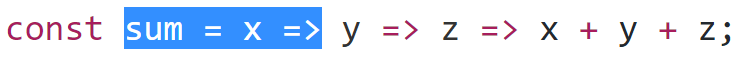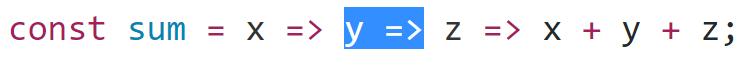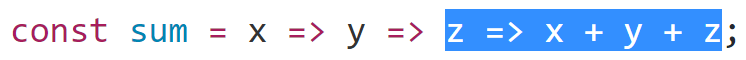## Currying

In mathematics and computer science, currying is the technique of translating the evaluation of a function that takes multiple arguments (or a tuple of arguments) into evaluating a sequence of functions, each with a single argument.

(source wikipedia)

## Prologue

Next time you read code that contains a sequence of arrow functions as in the following snippet, don’t freak out. You are not alone!

Yes, having a sequence or a chain of arrow functions is hard to read at first but there is a reason why its becoming more popular (checkout Redux applyMiddleware as an example) as it allows functional style goodness like passing curried functions to map/reduce functions. Let’s see this means and how we can read it.

## What currying means

Take the following simple sum function as an example. It takes three numeric arguments and returns the sum.

``````const sum = (x, y, z) => x + y + z;
console.log(sum(1, 5, 6));
// 12
``````

We can achieve the same result using this syntax (which is not using arrow functions):

``````var sum = function sum(x) {
return function (y) {
return function (z) {
return x + y + z;
};
};
};
console.log(sum(1)(5)(6));
// 12
``````

Now we have three nested functions each one is taking one argument and returning the following function that takes the next argument, except that last function which is doing the calculation.

## How to call

Since sum now only takes one argument: x, we can call it like this:

``````sum(1);
// [ Function]
``````

However we also know that it returns a function that takes y, so we can call it like this:

``````sum(1)(5);
// [ Function]
``````

And we know that that also return a function that takes z, so we can call it like this to return the sum of the three numbers:

``````sum(1)(5)(6);
// 12
``````

## Why is that useful?

We can now pass curried functions to other functions:

``````[1, 2, 3, 4].map(sum(1)(5));
// [ 7, 8, 9, 10 ]
``````

## Rewrite using arrow functions

Now we can also make use of arrow functions to make our functions more concise, and we can still achieve the same result.

``````const sum = x => y => z => x + y + z;
console.log(sum(1)(5)(6));
// 12
``````

1- sum is a function that takes “x” as an argument….2- and return a function that takes “y” as an argument…3- and return a function that takes “z” as and argument and returns the sum of “x”, “y” and “z”.First published on medium